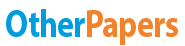# Data Analysis Normal Distribution

Essay by   •  June 3, 2017  •  Coursework  •  3,023 Words (13 Pages)  •  1,300 Views

## Essay Preview: Data Analysis Normal Distribution

Report this essay
Page 1 of 13
1. Calculate at least 2 measures of central tendency and at least 2 measures of variation for your Continuous Variable sample data. Explain the results and meaning of these measures in your report. Include a summary of the data in your report using a graph or table. Use Excel for this task.

Central Tendency

Central tendency is a single value that describes the  mean , median or mode in a set of data. Each of these measures have different calculation for different purposes. https://statistics.laerd.com/statistical-guides/measures-central-tendency-mean-mode-median.php

For example people want to compare their quiz scores to other students’ scores so the central tendency is important in comparing their score to the class distribution’s scores.

Mean

Also know as the average , this measure is the most widely used in central tendency for discrete and continuous variables. The mean value is obtained by dividing the sum of all the values in the data by the number of values in the data set.

However , the disadvantage in mean is that its values can be affected by outliers whose numerical values are either extremely small or large.

https://statistics.laerd.com/statistical-guides/measures-central-tendency-mean-mode-median.php

Mode

The mode is the most frequently occurring value.  It is used to uncover the most common occurring digit or category. For example , in a histogram , the tallest category will be the mode.  The mode is useful in categorical data , however , when used in continuous data such as interval and ratio scales , there may not have any data points of the same value due to decimal places so mode may not exist there.

http://www.quickmba.com/stats/centralten/

Median

The median is middle set of value obtained from an arrayed data set from lowest to highest values. The median is often used because it is not affected by skewness and outliers that could distort the mean . For example , when surveying the salary of a group of working adults , if the wages of two people are 5 times the mean , the unusually large salary will affect the mean salary making the values uneven and higher so in such a scenario , the median is a better representation of the group’s salary level.  http://www.quickmba.com/stats/centralten/

At least 2 measures of variation for your Continuous Variable sample data. (Standard deviation included in normal distribution paragraph.)

Skewness

Skewness determines how symmetrical is the distribution. A positive skew has higher values with a long tail to the right while a negative skew has lower values with a long tail to the left. A symmetrical distribution has a skewness of 0 which is important because it is used to identify normal distribution.

Kurtosis

Kurtosis measures the peak and whether the data is light-tailed or heavy-tailed when comparing with a normal distribution. A normal distribution can be identified with a kurtosis value of 0.

Positive and negative kurtosis deviates from the value 0. A positive kurtosis like data from a t distribution has postive values with a heavier tail and sharper peak compared to normal distribution like data. A negative kurtosis has negative values with a flatter peak and lighter tails when compared with normal distribution.

http://support.minitab.com/en-us/minitab/17/topic-library/basic-statistics-and-graphs/summary-statistics/how-skewness-and-kurtosis-affect-your-distribution/

Range

The range is the value obtained by deducting the highest score from the lowest score in a data set to find out how spread out is the data values are. A measure of the spread indicates if the mean is accurate in representing the data. If the spread of data is large, the mean is not useful to represent the data because a large spread means there are large differences between individual values. Thus, range comes hand in hand with mean to determine which measure is best to accurately interpret the statistics calculation.

Continuous Random Variable (CRV) CRV (table 1&2)

CRV can take on infinitely any values in an interval. And the CRV values are normally measured not counted so it tends to have decimals and is used to model characteristics like time, weight and length. http://dept.stat.lsa.umich.edu/~ionides/425/notes/continuous_rvs.pdf

Our CRV will be the length of time in the sauna to get a rough estimate of how long does each person stay in the sauna.

Q2. Discrete Random Variable DRV (TABLE 3&4) - determine the number of usage between M/F

Definition: A discrete variable is a variable that gets its value through counting distinct values (whole numbers) like 0,1,2,3,4 . http://www.stat.yale.edu/Courses/1997-98/101/ranvar.htm

Our discrete random variables will be the number of males and females and we would be using binomial distribution as explained below.

Binomial

A binomial distribution is a discrete probability distribution.

http://www.r-tutor.com/elementary-statistics/probability-distributions/binomial-distribution

This is an experiment with a fixed number of independent trials that has two mutually exclusive outcomes normally either success or failure. The probability of each outcome from trial to trial remains constant because it is independent. https://people.richland.edu/james/lecture/m170/ch06-bin.html  Independent means each trial has no effect on any of the rest. http://statistics.about.com/od/ProbHelpandTutorials/a/When-Do-You-Use-A-Binomial-Distribution.htm

Why use Binomial?

The binomial method is a specific probability distribution to estimate the percentage of success or failure in the outcome when counting our discrete variable. It is relevant in our experiment because we are determining the usage between males and females to calculate the success and failure rate of the saunas.

...

...